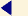back to menu
STRUCTURAL DESIGN - PART 016

English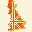26. Load combination with the flood force

The flood force has been applied to the bridge together with the permanent loads, assuming for both these loads a factor of 1.0, to the load combinations used in the F.E. model of the phase A.

The flood force was applied according to the steps and sub-steps listed below. The first and the second steps consist of one sub-step each, while the other steps were divided into two steps each.

 load force % Load Step Sub Step 100% Dead weight 1 1 Flood force C Q=1500 2 2 Flood force C Q=1750 3 4 Flood force C Q=2000 4 6 Flood force C Q=2250 5 8 Flood force C Q=2500 6 10

The flood force acts in the horizontal plane, in the weakest plane of the bridge.

The flood produces a total shear much higher than the shear produced by the seismic actions, but the stresses and strains are lower than those produced by the earthquake, because the flood force is concentrated prevalently on the lateral sides of the bridge.

The following figure shows the load-displacement diagram, obtained for the joints at the crown of the arch.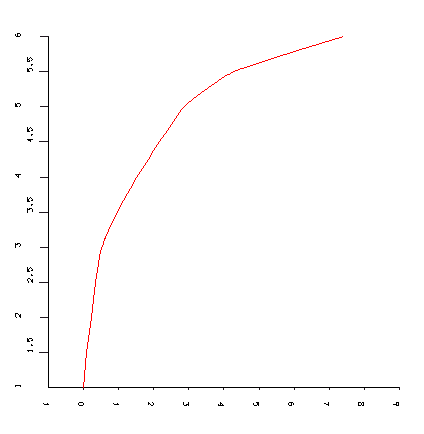displacements (horizontal axis) in the Z direction (in 10-1 cm)

Up to the load step no. 3 (flood of 1750 m3 / s) the bridge has a linear behaviour, later the overall stiffness reduces, with a hardening branch up to the load step no. 5 (flood of Q = 2250 m3 / s). The inclination of the diagram changes again and there is another branch of plastic-hardening up to the load step no. 6 (flood of 2500 m3 / s).

The following figures show the principal stresses S1, S2 and S3.

S3 Assonometric view at the load step no. 2 (Q = 1500 m3 / s)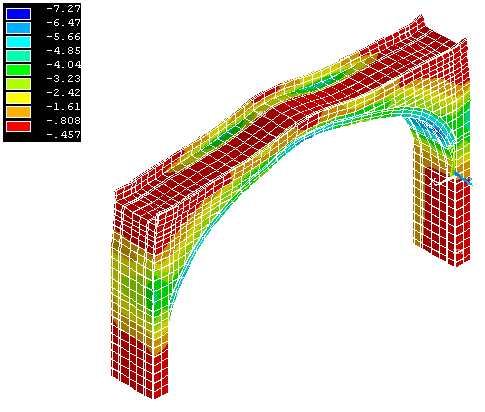S3 Assonometric view at the load step no. 3 (Q = 1750 m3 / s)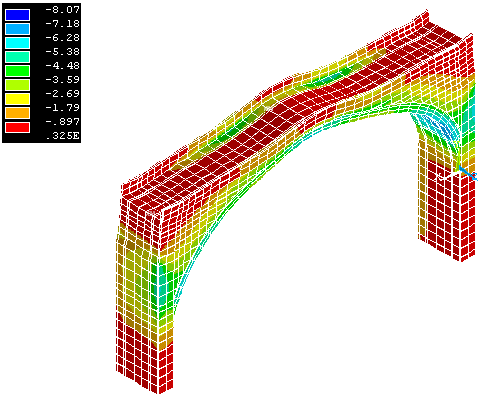S3 Assonometric view at the load step no. 4 (Q = 2000 m3 / s)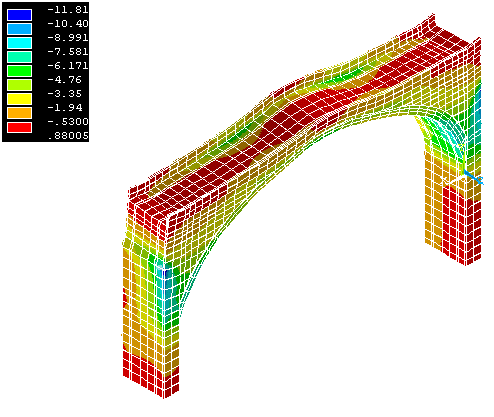S3 Assonometric view at the load step no. 5 (Q = 2250 m3 / s)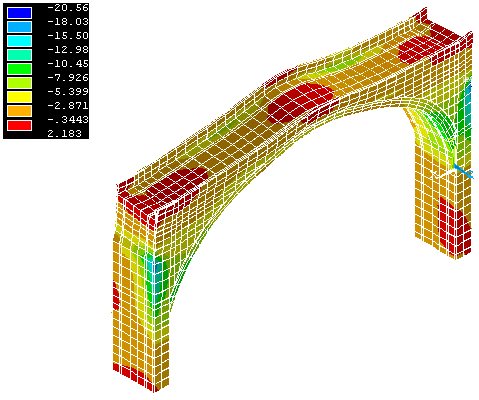S3 Assonometric view at the load step no. 6 (Q = 2500 m3 / s)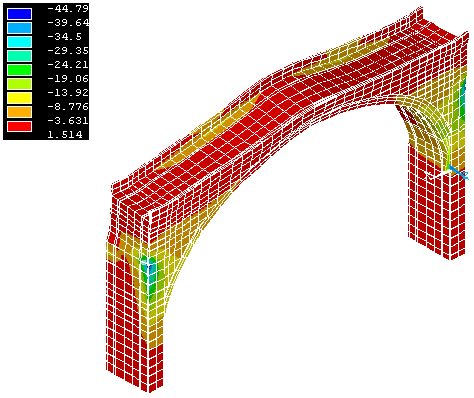S3 Assonometric view at the load step no. 6 (Q = 2500 m3 / s)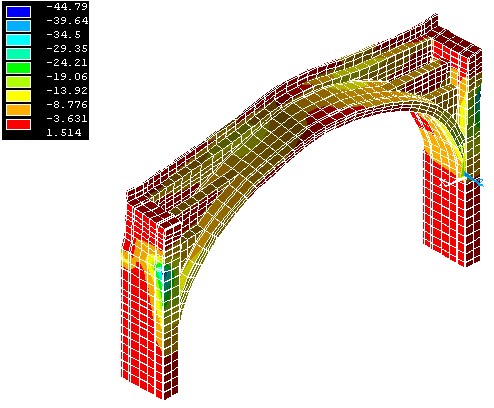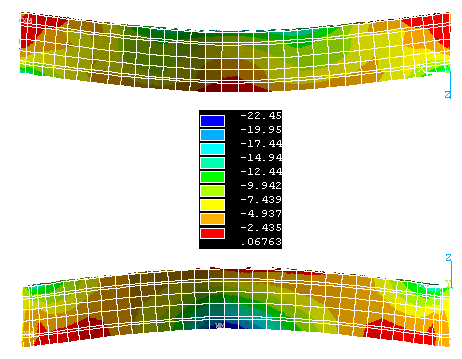The main results are:

the stresses and strains are not worrying up to Q = 2000 m3 / s; at the crown of the arch, the compression induced by the dead weight higher than the tractions induced by the flood. The tractions at the crown section arise only for Q = 2250 m3 / s.

The following figures show the principal stresses S2 and S1 (in Kg/cmq).

S2 Vista Estradosso (in alto) e Intradosso (in basso) al passo - Load Steps6 (Q = 2500 m3 / s)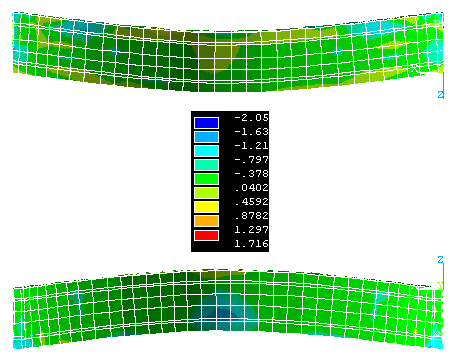S1 Vista Estradosso (in alto) e Intradosso (in basso) al passo - Load Steps6 (Q = 2500 m3 / s)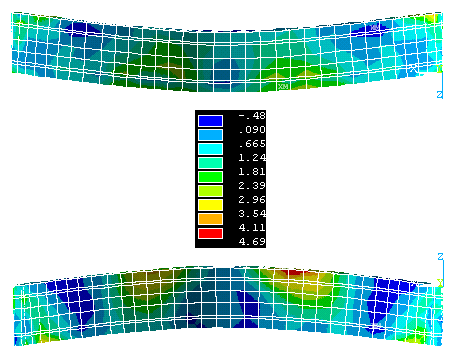CREDITS:

Intellectual property of this report and of the design drawings is owned by the University of Florence - Department of Civil Engineering

author of the text: Prof.Eng. Andrea Vignoli – other contributes have been mentioned in related paragraphs

© - General Engineering Workgroup -

SOURCE:

Final Design Reportback to menu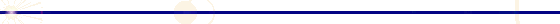GENERAL ENGINEERING - P.zzale Donatello 4 - 50132 Firenze - Italy - ph. +39 055 2345256 - fax. +39 055 2476074